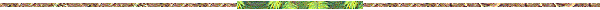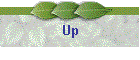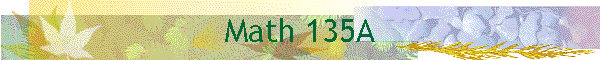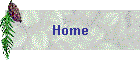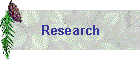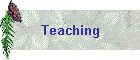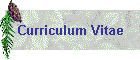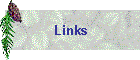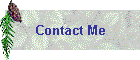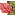Course: Math 135A, Ordinary Differential EquationsText: G. Simmons, Differential Equations with Applications and Historical Notes, 2nd Edition, McGraw HillSchedule of Lectures: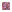Section 1-4: General remarks on solutions, families of curves, orthogonal trajectoriesSection 5: Motion problemsSection 6: The brachistochrone, treated without calculus of variationsSection 7-11: Homogenous and exact equations, integrating factors, linear first order equations, reduction of orderSection 12: The catenary, assign 13 as reading (review of RLC circuits)Section 14-16: Second order linear equations, homogeneous equations, the use of a known solution to find anotherSection 17: Homogeneous equations with constant coefficientsSection 18: The method  of undetermined coefficientsSection 19: The method of variation of parametersSection 20: Vibrations in mechanical and electrical systemsSection 21: Newton's law of gravitation and the motion of the planetsSection 22: Higher order linear equations, coupled harmonic oscillatorsSection 23: Operators methods for finding particular solutionsSection 24-25: Oscillations and the Sturm separation theorem, Sturm comparison theoremSection 27-31: Review of power series, series solutions of first order equations, second order equations, ordinary points, regular singular points, Gauss's hypergeometric equation.